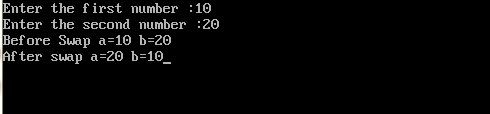UncleCoder.com

UncleCoder.com

Free programming examples and instructions

# C program to swap two numbers without using third variable

## c program for how to swap two numbers without using third variable

by Krishna

Posted on 27 Jul 2018 Category: C Views: 1118

C program to swap two numbers without using third variable

We can swap two numbers with and without using a third variable. Let's see a simple C program to swap two numbers without using third variable. Source code for the same is given below.

``````#include<stdio.h>
#include<conio.h>
void main()
{
int a,b;
printf("Enter the first number :");
scanf("%d",&a);
printf("Enter the second number :");
scanf("%d",&b);
printf("Before swap a=%d b=%d",a,b);
a=a+b;//a=30 (10+20)
b=a-b;//b=10 (30-20)
a=a-b;//a=20 (30-10)
printf("\nAfter swap a=%d b=%d",a,b);
getch();
}   ``````

OUTPUT: